# How Can You Contribute to the World With Data Structures and Algorithms?

By Sonalisha Mohanty Category Full Stack Reading time 12.6 mins Published on Sep 19, 2023

Learn Data Structures and Algorithms Course to Have a Social Impact!!

The digital revolution has redefined many business entities and associated operations with its tremendous innovations. Such tech creations have transformed many sectors with their profound presence, paving the way for a promising future. Amidst such changes, data-driven culture has resurfaced in the business world with advancing technologies that one should not miss. To lead and manage the data-driven culture of the firms, experts like coders, programmers, & developers are making their way. And do you know what’s the magic skill leading all kinds of success in this regard? It’s data structure and algorithms.

Undoubtedly, future courses of action will depend on programmers & developers to reach sustainable solutions. Yet, persisting skill gaps can deviate them from reaching their goals. Thus, a sound knowledge of data structures and algorithms must be developed to face the competitive future. Upskilling with a proven data structures and algorithms course can dismiss the hurdles on the way toward skills development.

Programming is everywhere. A skilled programmer is required everywhere, from breaking down data patterns to extracting insights. Hence, potential knowledge of DSA concepts can improve coding skills for generating real-time gains. As mentioned earlier, consistent learning with a data structures and algorithms course online can pave the way for efficient coding skills.

Do you know a knowledge of DSA can influence society and its well-being in a positive way? In this blog, you will explore the notable contributions made by data structures and especially algorithms that leave an insightful imprint on society.

Before that, let us understand algorithms.

## The Algorithms in Brief

An algorithm is a vital part of DSA that helps the developers or coders with a set of instructions to resolve a problem. Since its evolution, the term algorithm has been witnessed in each field. Algorithms are useful in various ways, from decoding an attacker’s next move to making job-related decisions. In the computer science stream, the term algorithm is widely used, and it helps the users to solve any problem with a few steps. Anyone who is upskilled with a data structures and algorithms course can experience these uses in real-time.

The system reads the concern presented by the user and processes it with the use of algorithms. After this step is completed, it generates instructions that the user must follow to solve their problem. Algorithms use various tools like natural language processing, programming languages, and flow charts in order to decode and transmit accurate information. The experts upskilled through a data structures and algorithms course online can work with these tools and reach a solution.

The ancient years and certain instances are clear evidence of real-time uses of algorithms in the world. Let us know more.

## Algorithm in Empowering Human Survival

Data structures and algorithms have profound potential to control and safeguard many lives. Our rich history unfolds many such instances where an effective use of algorithms has changed the future of mankind. Algorithms have been buzzing frequently in recent times due to the fast-paced rise of tech shifts taking place around the world. Hence, gaining a proven knowledge of DSA has become essential for every tech professional. Gaining hands-on experience with a data structures and algorithms course can increase the chances of success.

If we dive deep into the past, algorithms are nothing new. Its presence was felt years ago; on the contrary, an effective use is present. The rapid evolution of algorithms since the ancient days has made them expensive today. Yet, the new age of the algorithmic approach reflects on past instances. Learning the latest trends through a data structures and algorithms course online helps justify the essence of algorithms. Let us know more about it.

The algorithm is pervasive and its influential presence all over the world is felt by the users. In the past, during World War II, a proven use of algorithms entails its significance in saving millions of lives. Algorithms have been there since the 19th Century, and their modern yet relevant use in recent years has changed the notion.

In World War II, algorithms were used as a communication medium with the troops. The basic knowledge of Morse code helped the warriors communicate with their teams. Basically, during a war, encrypted messages are shared with the team members to safeguard themselves from danger. Yet, decoding each message was hard. Then, a decoding machine was introduced by Alan Turing that applied the knowledge of data structures and algorithms that cracked the message.

The past war instance here proves the efficient use of algorithms in cracking down on the right message and safeguarding the teams. At present, if you are upskilled with an industry-paced data structures and algorithms course, then career success is assured.

Proficiency in writing codes helps the developers decode the real info and solve the problems encountered. This is why upskilling with a data structures and algorithms course online is essential for tech enthusiasts. But how to write efficient code in real time?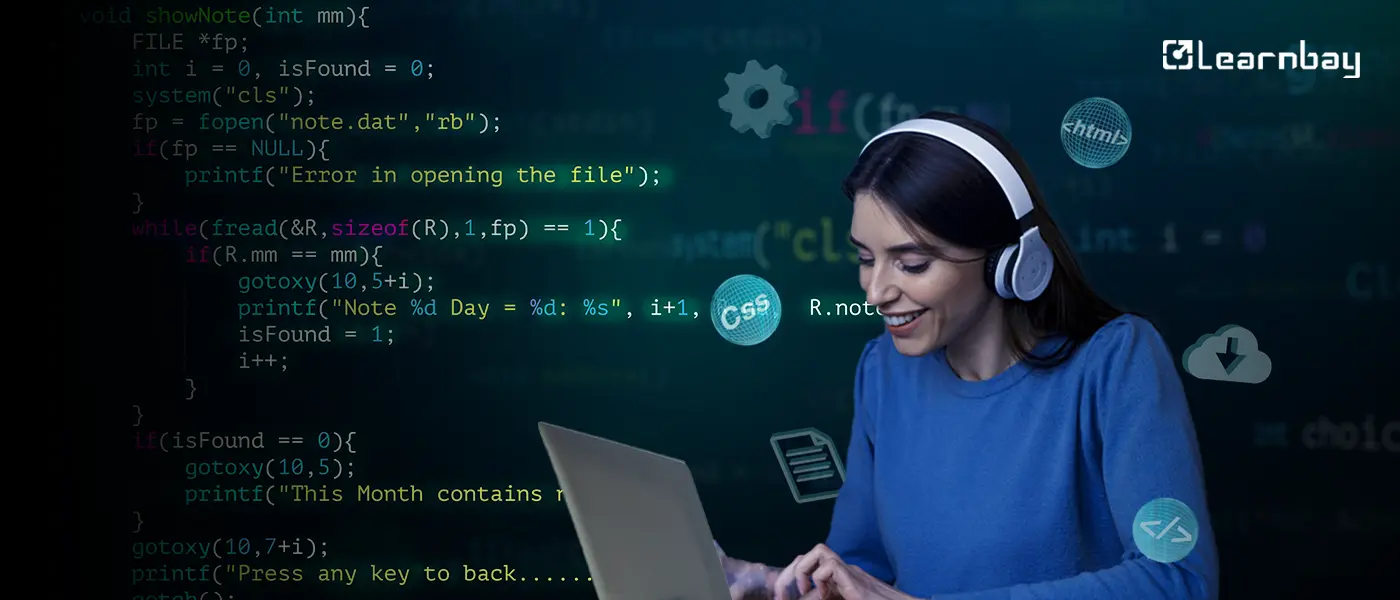## Learn & Master Efficient Coding Skills with DSA

An industry-paced DSA program helps tech experts master coding skills with the inclusiveness of algorithmic thinking. The use of algorithms elevates the level of coding expertise that helps the experts decode the inputs well. After learning DSA, a tech expert will be able to work well with various data structure types and algorithmic models.

Various types of algorithms provide good or bad results. Yet, choosing the right one requires proven DSA skills and practical expertise. Learning through a data structures and algorithms course can leverage the skills to deal with practical cases.

When you enroll in an industry-paced DSA program, gaining hands-on experience in different areas becomes easy. With the practical learning of tools and techniques in DSA, an expert can level up his coding skills and ease the message-decoding process. Consistent learning with a proven data structures and algorithms course will give you benefits like -

• Improvement in programming expertise

• Optimized codes generated with fewer or no bugs

• Software with fast-running operations

A tech enthusiast eager to shape a career in software development or engineering must be efficient in coding. Basic knowledge of DSA concepts and uses in real-time can help in software development-related job roles.

## How to Refine Your Programming Expertise?

Improving programming skills is vital for shaping a tech career in this digital era. Upskilling through a data structures and algorithms course online can help you strengthen your coding skills. Yet, refining coding skills will require ‘measuring the level or efficiency of the code’ at first. Measuring the coding efficiency can help the experts identify and address the loopholes Anyway, this will require an intervention of time complexity.

### Time Complexity

An instance of the time complexity of search algorithms is as follows:

Let's take a detailed look at the example below to obtain more knowledge about the time complexity of search algorithms. In this section, we'll contrast two distinct problem-solving methods. The issue that we shall discuss now is being researched.

Let's imagine that we need to find a certain element in an array that has been organized in ascending order. We now have two algorithms for addressing this issue:

1. Linear Search.

2. Binary Search

Consider an array of five items, and where we need to locate the number 24 in the array.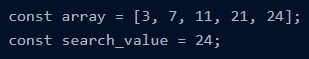Fig1: Locate the number in a constant array

The linear search method will now compare each member of the array to a certain search_value when we search. Now, it must return true if the search_value is identified. That seems easy, right? If not, practical learning with a data structures and algorithms course online can make it easy.

Let's now check the total number of operations required for it to return its result. Since it compares each member of the array individually, we arrive at number 5 as the response. We can now state that the provided element is found using linear search using a maximum of five operations. In the situation of linear search, this is typically the worst possible scenario for an algorithm.

To summarize, in the worst scenario, the linear search requires 'n' operations (where 'n' is represented as array size).

Let's now look at the Binary search method in detail using an easy example.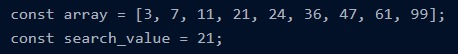Fig 2: Binary Search Method

Now for the provided array, if we had to implement the binary search method to identify the search_value we would start by looking in the center of the array. Now that the array's center element is 24, we analyze to see whether 24 > 21, so we start looking left by looking at the items to the left. We notice that we will discover a number, say 7, and since 7 < 21, we start looking for the desired element, and once we reach 21, we compare 21 = 21.

When we count the number of steps, the binary search required to identify the target element, we realize that it took four rounds. This demonstrates why the binary search utilizes a logarithmic technique.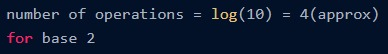Fig 3: Binary search (2)

When using a binary search technique, the standard form is expressed as

The number of steps executed by the Binary Search for an array of size n is: log(n)

### Asymptotic Analysis

#### Big-O Notation

Big-O notation refers to the expression of the maximum running time allowed for an algorithm. Thus, it reflects the worst-case complexity. Further knowledge can be gained if you enroll in a data structures and algorithms course online.

Here, ‘O’ in Big-O Notation refers to the order of a function.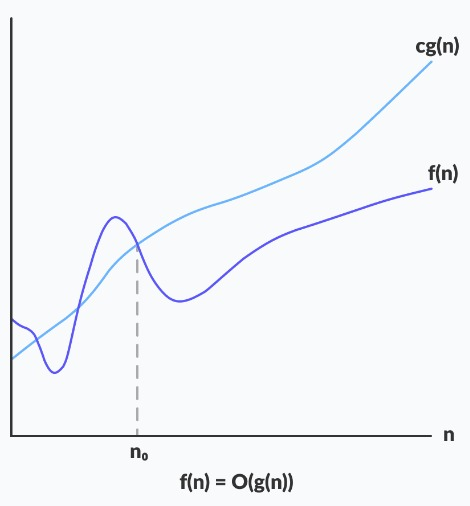Here, cg(n): positive constant

f(n): function

Fig 4: Big O Notation Graph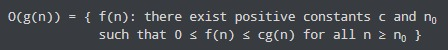Fig 5: Big O Notation Graph Interpretation

The statement above could be expressed as a function f(n) about the set O(g(n)) if a positive constant c is found that stands between 0 and cg(n), for enough big n.

Take a random value for n, the duration of the execution of an algorithm never exceeds the time given by O(g(n).

Since we are always keen on the worst-case situation, it is frequently used to examine an algorithm since it provides the worst-case execution time of an algorithm. To know better, you must enroll in a data structures and algorithms course online.

Example 1:

Calculate the upper bound time of execution of the constant function f(n) = 6993.

We must identify c and n0 such that 0 ≤ f (n) ≤ c.g(n) for every n ≥ n0 to identify the upper bound of f(n).

0 ≤ f (n) ≤ c × g (n)

0 ≤ 6993 ≤ c × g (n)

0 ≤ 6993 ≤ 6993 x 1

So, c = 6993 and g(n) = 1

The above inequalities are satisfied by any value of c bigger than 6993, making all such values of c feasible.

0 ≤ 6993 ≤ 8000 x 1 → true

0 ≤ 6993 ≤ 10500 x 1 → true

The constant function f(n) does not change with the size n of the problem, So n0= 1

f(n) = O(g(n)) = O(1) for c = 6993, n0 = 1

f(n) = O(g(n)) = O(1) for c = 8000, n0 = 1 and so on.

Example 2:

Identify the upper limit of the running time of a linear function f(n) = 6n + 3.

To identify the upper bound of f(n) We must locate c and n0 such that 0 ≤ f (n) ≤ c x g (n) for every n≥n0.

0 ≤ f (n) ≤ c × g (n)

0 ≤ 6n + 3 ≤ c × g (n)

0 ≤ 6n + 3 ≤ 6n + 3n, for all n ≥ 1 (There are such endless possibilities)

0 ≤ 6n + 3 ≤ 9n

So, c = 9 and g (n) = n, n0 = 1

Tabular Approach

0 ≤ 6n + 3 ≤ c × g (n)

0 ≤ 6n + 3 ≤ 7 n

Now, manually identify the correct n0, such that f (n) ≤ c.g (n)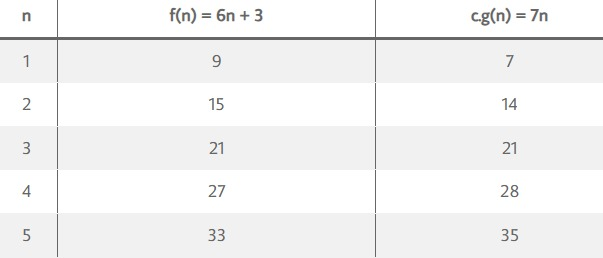Fig 6: Tabular Approach

According to the table, for n ≥ 3, f (n) ≤ c × g (n) holds true. So, c = 7, g(n) = n, and n0 = 3, There could be such multiple pairs of (c, n0)

f(n) = O(g(n)) = O(n) for c = 9, n0 = 1

f(n) = O(g(n)) = O(n) for c = 7, n0 = 3

and so on.

The learners with proven skills after learning through a data structures and algorithms course online, can solve these problems.

### Omega Notation (Ω-notation)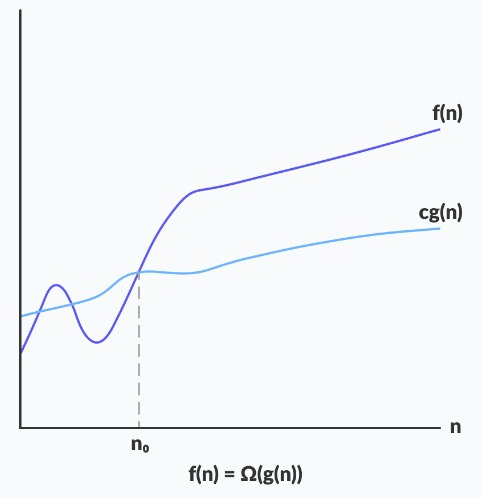Fig 7: Omega Notation Graph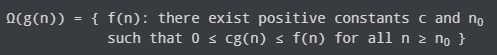Fig 8: Omega Notation Graph Interpretation

The statement above could be interpreted as a function f(n) about the set Ω(g(n)) if there is a positive constant c that stands above cg(n) for sufficiently big n.

The algorithm's lowest execution time is provided by Ω(g(n)) for any value of n.

Example:

Identify the lower limit of the time of execution of linear function f(n) = 6n + 3.

To determine the lower limit of f(n), we must identify c and n0 so that 0 ≤ c.g(n) ≤ f(n) for all n ≥ n0.

0 ≤ c × g(n) ≤ f(n)

0 ≤ c × g(n) ≤ 6n + 3

0 ≤ 6n ≤ 6n + 3 → true, for all n ≥ n0

0 ≤ 5n ≤ 6n + 3 → true, for all n ≥ n0

Both of the above discrepancies are true, and there are never-ending inequalities. So,

f(n) = Ω (g(n)) = Ω (n) for c = 6, n0 = 1

f(n) = Ω (g(n)) = Ω (n) for c = 5, n0 = 1

and so on.

After getting upskilled through a data structures and algorithms course online, you will be able to solve these tasks well.

### Theta Notation (Θ-notation)

The above as well as below functions are included in theta notation. Since it reflects the upper and lower limits of the duration of execution of an algorithm, it is utilized to check the average-case complexity of an algorithm.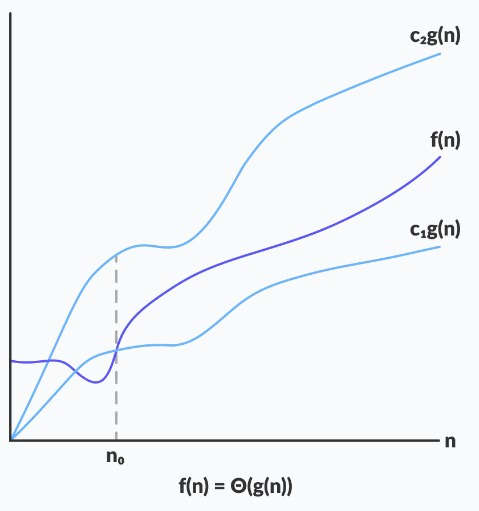Fig 9: Theta Notation Graph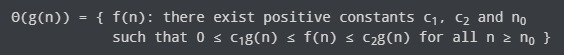Fig 10: Theta Notation Graph Interpretation

The above equation could be said that the function f(n) belongs to the set Θ(g(n)) If there are positive constants c1 and c2 that are positioned between c1g(n) and c2g(n), for an adequate big n.

A function is considered to be an asymptotically tight limit if it lies somewhere between c1g(n) as well as c2g(n) for every n≥ n0.

Example:

Identify the tight limit of the execution time of constant function f(n) = 23.

To identify the tight limit of f(n), we need to identify c1, c2, and n0 so that, 0 ≤ c1× g(n) ≤ f(n) ≤ c2 × g(n) for all n ≥ n0

0 ≤ c1× g(n) ≤ 23 ≤ c2 × g(n)

0 ≤ 22 ×1 ≤ 23 ≤ 24 × 1, → true for all n ≥ 1

0 ≤ 10 ×1 ≤ 23 ≤ 50 × 1, → true for all n ≥ 1

Both of the above inequalities are indicated true, and there exist never-ending inequalities.

So, (c1, c2) = (22, 24) and g(n) = 1, for all n ≥ 1

(c1, c2) = (10, 50) and g(n) = 1, for all n ≥ 1

f(n) = Θ (g (n)) = Θ (1) for c1 = 22, c2 = 24, n0 = 1

f(n) = Θ (g (n)) = Θ (1) for c1 = 10, c2 = 50, n0 = 1

and so on.

## End Notes

In conclusion, data structures, and algorithms are fundamentals in CS. Programming has become one of the trending skills in the tech industry, paving the way toward a digital future. The software development field deals with complex cases and a lack of relevant knowledge and skills can degrade the yields. Thus, the working pros must go through the right upskilling ways to refine their skills and sustain themselves in the field. Learning through an industry-curated data structures and algorithms course can be helpful in several ways.

Data Structures Algorithms and System Design is an industry-paced program supporting the career transition goal of tech enthusiasts. Its open learning platform and experience labs help the experts learn DSA concepts and tools in real-time. The professionals in the tech industry willing to refine their skills and retain their talent across the MNCs can opt for this program with assured results. Gaining practical experience with live projects and learning real use of the tools is possible with this course.

The placement assistance program highlights the USP of the program, which is the ‘career service pro’ package with a Flexi subscription. This package includes 1:1 mock interviews, resume-building sessions, 1:1 doubt-clearing assistance, & unlimited interview calls from industry partners. Plus, a globally recognized certification from IBM & Microsoft levels up the chances of getting hired across leading MNCs.

## FAQs:-

### Is learning DSA without programming skills possible?

No. DSA deals with understanding the problem and then solving it. Hence, before learning DSA concepts, one must know the in-demand programming languages. This will help them identify and analyze the depth of the concern and solve it in real time.

### Are DSA skills essential for cracking job interviews?

The product-based interviews in the tech industries are demanding for efficient coding skills. With the increasing search for skilled programmers, the need for DSA skills has increased. If you wish to retain your successful career in the tech field, upskilling with DSA is helpful.

### Is learning DSA hard for working professionals?

DSA consists of critical models and algorithmic thinking skills that often make the learning process longer and more difficult. The tech enthusiasts looking for upskilling must go for an industry-paced DSA program to have practical learning and experience with the related tools.